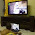## Sunday, January 16, 2011

### Arduino Pins - Analog Pins and Analog Reference Voltage

Arduino have 6 analog pins labeled as Analog 0 to 5. Analog pins are mostly used to read analog sensors and sent to the 6 channels Analog Digital Converter (ADC). The ADC will convert the voltage (0V to 5V) to integers from 0 to 1023 (10 bit resolution). This can be accomplished using the analogRead() function. If the analog sensors have a narrow voltage range, you can use the analogReference() to change the input range and resolution.

The analogReference() can be change from the default analog reference of 5V ( or 3.3V for some boards ) to either an INTERNAL analog reference or EXTERNAL analog reference.

Internal analog reference will use the internal 1.1V when converting from voltage of integer of 0 - 1023. If the AREF (Analog Reference)  pins is connected, it will USE the external reference voltage even if you issue the command analogReference(INTERNAL);

External analog reference will use the external AREF pin and take the reference voltage of whatever voltage that pins is connected to. You can use this method with a voltage divider to get 2.5V for the analog reference voltage. An example is to connect two resistors of same value (example 100 ohm each) to the 5V pin and tap in the middle to get the 2.5V into the AREF pin.  As normal resistor have a 5% tolerance value, it is not very accurate unless you use metal-film resistors with 1% tolerance value for better accuracy.

All the 6 analog pin can be used as General Purpose Input/Output (GPIO) pins like digital pin using label of A0 to A5. Use the command, pinMode(A0, OUTPUT) to set the analog pin to OUTPUT mode. After the mode is set to OUTPUT, you can use the digitalWrite() functions for these pins.

*** analogRead will not work correctly when set to OUTPUT due to the internal pull-up resistor. You need to use the pinMode to set it back to INPUT before using the analogRead() function.

1.Thanks particularly for explaining the analogReference(INTERNAL) command to force use of 1.1v on a UNO.

2.thnx.for the informatiom of internal reference voltage

3.I am trying to use the uno for reading in an accelerometer shock measurement. The accelerometer outputs 10mV/G experienced. The accelerometer data sheet says that this is an Ac voltage output. I've read in the accelerometer output using an oscilloscope and i've connected a AA battery to the analog pin in which i'm using for the accelerometer. When the AA battery is connected I get 155G which is correct because the battery outputs 1.5V. However when i connect the accelerometer i get nothing at all.
Any suggestions would be greatly appreciated

4.Pls join me facebook group and do post the links to yr acceleromoter datasheets there so that other members can help you too...

5.can analog pin do on off like digital pin??
if have can show the code...

6.